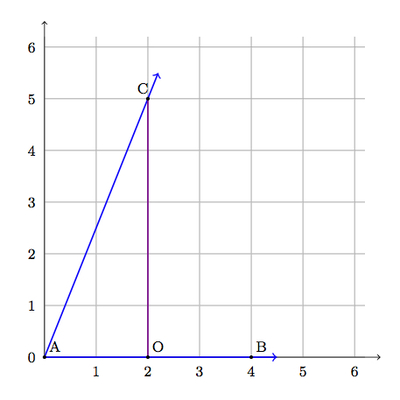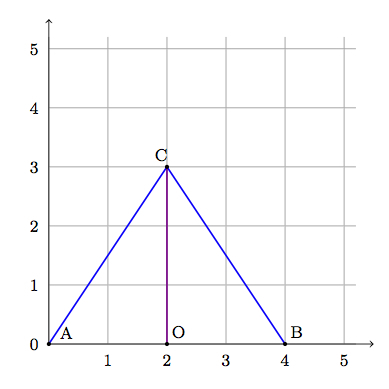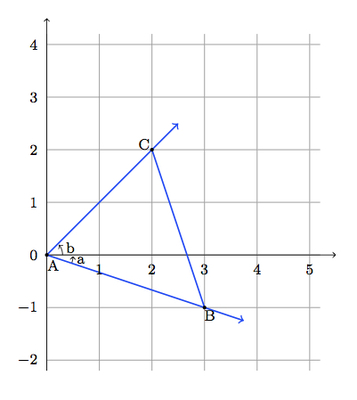# Coordinates of equilateral triangles

Alignments to Content Standards: G-GPE.B N-RN.B F-TF.C.9

Is there an equilateral triangle $ABC$ so that $\overline{AB}$ lies on the $x$-axis and $A$, $B$, and $C$ all have integer $x$ and $y$ coordinates? Explain.

## IM Commentary

The purpose of this task is to examine angles of triangles whose vertices have specific integer coordinates. The angles examined are formed by three points with integer coordinates, two of which are on the $x$-axis. There are many such angles as the only constraint on the non-horizontal ray is that it belongs to a line with rational slope. It turns out that the impact of that slope being rational is that it makes a 60 degree angle, with these conditions, impossible, and so no equilateral triangle exists satisfying these constraints. Students will need to either know or be able to calculate the length of the altitude of an equilateral triangle or know the sine and cosine of a 60 degree angle. This can be done via knowledge of trigonometric functions or with the Pythagorean Theorem. The teacher may need to provide guidance: a good starting point would be to draw some triangles, as in the solution, and determine whether or not these special cases are equilateral.

The task can be naturally extended to show that there is no equilateral triangle in the plane whose vertices have integer coordinates: in other words, the answer to the problem is the same without the assumption that two of the vertices are on the $x$-axis. An argument for this more general problem is presented using sum and difference formulas for the tangent function. This is a plus standard and the formulas will likely need to be given. It is not the precise formulas themselves, however, but rather their structure which is vital: this task can be viewed not only as an application of these formulas but also a motivation to investigate them.

The idea for this task came from an Albuquerque Math Teachers' Circle meeting on October 1, 2013.

## Solutions

Solution: 1 Trigonometric Functions

Suppose there is an equilateral triangle $ABC$ satisfying the given hypotheses. We can make a horizontal translation so that $A = (0,0)$ and we will assume, for simplicity, that $B$ lies on the positive $x$-axis. Below is a picture of an example where the $y$-coordinate of $C$ is positive:Suppose $m(\angle A) = 60$. We can calculate the trigonometric functions for $\angle A$ by making a right triangle as pictured below:Since the grid lines are perpendicular $\angle O$ in $\triangle AOC$ is a right angle and we have $$\tan(\angle A) = \frac{|OC|}{|AO|}.$$ For the particular triangle we have drawn, $|OC| = 5$ and $|AO| = 2$ but in general if $C$ has integer coordinates (a,b) then we will get a rational number $\frac{b}{a}$ for the value of $\tan(\angle A)$. Since $\tan(60) = \sqrt{3}$ and $\sqrt{3}$ is an irrational number, this means that $m(\angle BAC)$ cannot be 60. The fraction $\frac{b}{a}$ giving the value of $\tan{\angle A}$ only makes sense when $a \neq 0$ but if $a = 0$ then $\angle A$ would be a right angle and $\triangle ABC$ would still not be equilateral. Therefore there is no equilateral triangle $ABC$ with two vertices on the $x$-axis and all vertices having integer valued coordinates.

Solution: 2 Pythagorean Theorem

Suppose there is an equilateral triangle $ABC$ satisfying the given hypotheses. We can make a horizontal translation so that $A = (0,0)$ and we will assume, for simplicity, that $B$ lies on the positive $x$-axis. In the picture $\overleftrightarrow{CO}$ is perpendicular to $\overleftrightarrow{AB}$.If the triangle were equilateral then $2|AO| = |AC|$. Since $\triangle AOC$ is a right triangle, the Pythagorean Theorem says that $$|OC|^2 + |AO|^2 = |AC|^2.$$ Substituting in $|AC| = 2|AO|$ means that $|OC|^2 = 3|AO|^2$ and hence $$\sqrt{3}|AO| = |OC|$$ and $\sqrt{3} = \frac{|OC|}{|AO|}$. If $\triangle ABC$ were equilateral then $|AC| = |AB|$ and $|AO| = \frac{|AB|}{2}$ are rational numbers. This would mean that $\sqrt{3}$ is a rational number. This is not true, however, and so $\triangle ABC$ cannot be equilateral.

Solution: 3 Addition Formulas for Trigonometric Functions (F-TF.9)

This solution builds on the first solution using trigonometry. The goal here is to use the sum/difference formula for the tangent function which says that $$\tan{(a \pm b)} = \frac{\tan{a} \pm \tan{b}}{1 \mp \tan{a}\tan{b}}.$$ Recall that $\tan{x} = \frac{\sin{x}}{\cos{x}}$ and this only makes sense when $\cos{x} \neq 0$. So we must make sure in our argument below that $a, b,$ and $a \pm b$ are not $\pm 90$. Note also that the different signs of the tangent expressions in the numerator and denominator.

Below is a picture showing a specific triangle to which we can apply the sum formula given above:In this case we have $m(\angle A) = a + b$ and, using the formula above

\begin{align} \tan{\angle A} &= \tan{(a + b)} \\ &= \frac{\tan{a} + \tan{b}}{1 - \tan{a}\tan{b}}. \end{align}

As seen in the first solution, both $\tan{a}$ and $\tan{b}$ are rational numbers: in this case, $\tan{a} = \frac{1}{3}$ and $\tan{b} = \frac{2}{2}$. Using the formula above we find that $\tan{\angle A}$ is a rational number. On the other hand, if $m(\angle A) = 60$ then $\tan{A} = \sqrt{3}$, an irrational number. So it is not possible for $m(\angle A) = 60$ and it is not possible that $\triangle ABC$ is an equilateral triangle.

Any triangle whose vertices have integer coordinates can be translated so that one of the vertices, say $A$, is at (0,0) and the other two vertices have integer coordinates. We can also apply 90 degree rotations about the origin (which map integer coordinates to integer coordinates) so that one of the other vertices, which we label $C$, lies in the first quadrant and $m(\angle BAC) \gt 0$. The only way the triangle can be equilateral is if the third vertex $B$ lies either in the fourth quadrant or in the first quadrant. The case where $B$ lies in the fourth quadrant has been handled above (the denominator $1 - \tan{a}\tan{b}$ can not be zero if $\angle A$ is a 60 degree angle). If $B$ lies in the first quadrant then a similar argument using the formula for the tangent of the difference of two angles applies. A picture of this other situation is given below: